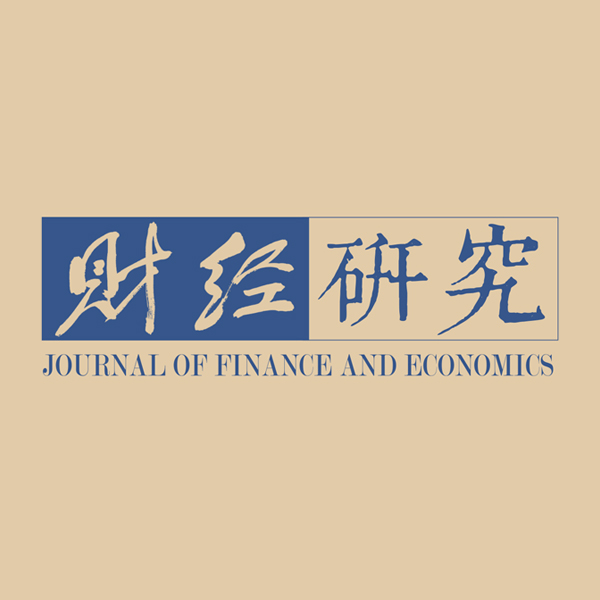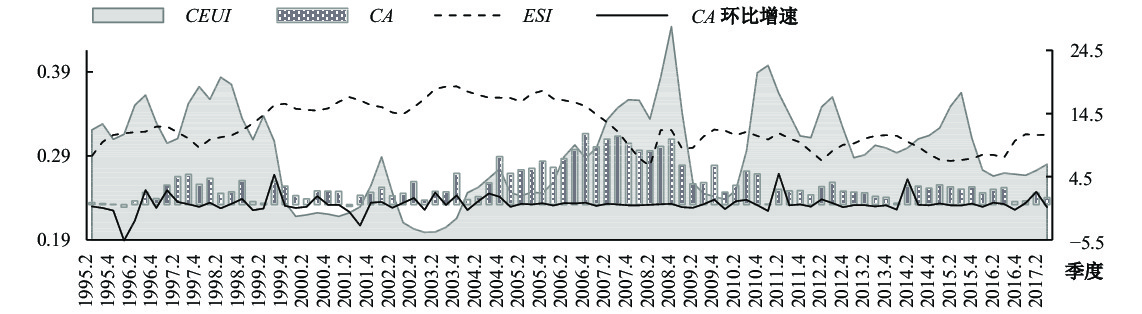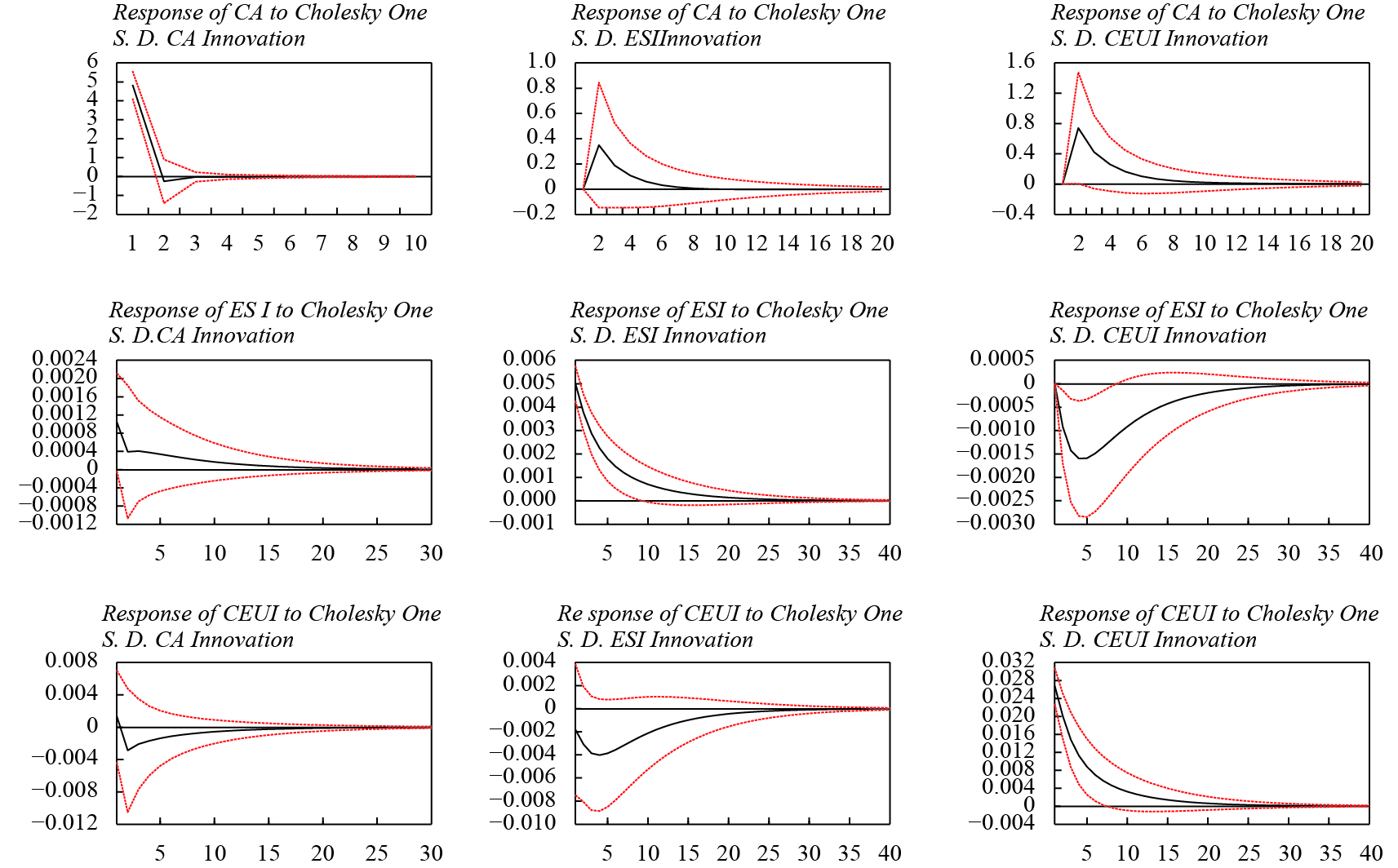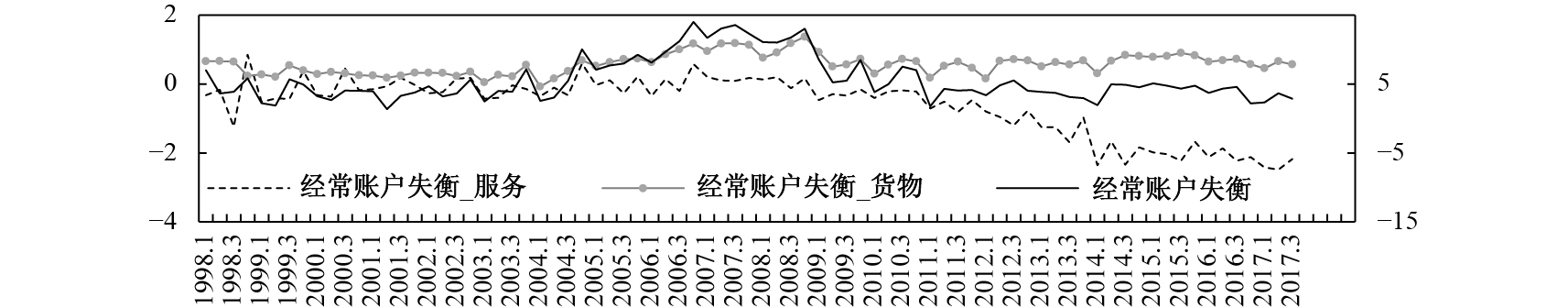﻿ 国际经济协作、国内经济稳定与经常账户失衡改善《财经研究》
2019第45卷第8期

International Economic Cooperation，Domestic Economic Stability and the Improvement of Current Account Imbalances
Ma Dan, He Yaxing, Li Chun
School of Statistics，Southwestern University of Finance and Economics，Chengdu 611130，China
Summary: After 2008, the barriers to cross-border flows of production factors such as commodities, capital, and labor have become increasingly apparent. The new round of liberalism has quietly risen, triggering a wave of counter-globalization characterized by " anti-free trade and anti-integration”. Some developed countries have attempted to go beyond the constraints of the structural adjustment of the global economy and embark on the so-called " priority of interest and self-development”. This, to a certain extent, weakened the synchronization of the world economic cycle and objectively exacerbated the " synthetic fallacy” in the global economic development. In the absence of effective international economic rules and regulations, this " synthetic fallacy” may cause frictions in trade, finance, and other areas to increase, thereby accelerating current account imbalances. Based on the above facts, this paper regards current account imbalances as the result of the internal and external factors, and combines the changes in the economic globalization process with macroeconomic uncertainty to explore whether the synchronization between China and the world economic cycle has changed. Is there any uncertainty in China’s macro-economy, and how does it affect current account imbalances, accelerating or smoothing? If the synchronization between China and the world economic cycle is reduced and macroeconomic uncertainty is raised, will it accelerate current account imbalances? What is the impact? Firstly, the method of real-time measurement of macroeconomic uncertainty using large-scale mixed macroeconomic data sets is proposed, and the macroeconomic uncertainty index is constructed. It avoids the possibility of directly using the substitution variables such as financial market volatility in the past literature to reflect the possible deviation of macroeconomic uncertainty. Then we use the Markov framework to establish a macroeconomic cycle synchronization index. Finally, the vector autoregressive model with Bayesian variable selection （VAR-BVS） weakens the endogeneity problem caused by missing or mistaken variables, and analyzes the dynamic relationship between the three before and after the global financial crisis. The study examines the possible links between the three. The study finds that: The relationship between the two is similar to a U-shape curve with the synchronization of the world economic cycle as the horizontal axis and current account imbalances growth rate as the vertical axis. Whether before or after the crisis, macroeconomic uncertainty plays an " accelerator” role in current account imbalances. In the long term, the increase in macroeconomic uncertainty has an accelerating effect on current account imbalances. In the short term, in addition to the impact of the domestic economic environment, the decline in the synchronization of the world economic cycle will play an important role in accelerating current account imbalances. The reason for the synchronization between China and the world economic cycle, and macroeconomic uncertainty affecting current account imbalances may be due to the international division of labor, factor mobility, and preventive savings motivation. There is structural heterogeneity in the relationship between domestic and foreign economic environment and current account imbalances, which is different from the relationship between trade in goods and trade in services. The findings show that a stable domestic economic environment and a cooperative international economic environment are important conditions to improve current account imbalances.
Key words: synchronization of the economic cycle    macroeconomic uncertainty    current account imbalances

（一）指标设计

（二）指标测算

1. 宏观经济不确定性的测算。在测算宏观经济不确定性时需考虑投资、生产、物价、金融等多方面因素。估计不确定性时面临三个重大挑战：一是宏观经济指标较多，大型宏观经济数据之间存在协同变动（Stock和Watson，1989，1991）；二是在实际问题中，所有指标并不是完全同频率；三是指标过多，重要变量的贡献易被重要性较低的变量稀释，导致估计结果偏差。基于这些挑战，本文建立了含有潜在不可观测变量的混频动态因子随机波动模型（Mixed_GFSV model），将宏观经济不确定性表示为两部分的加权和：第一部分为各指标之间的公共波动，反映指标间共同的不确定性；第二部分为各指标的异质波动，反映指标本身的不确定性。

 \begin{aligned} & {y_t} = \lambda {F_t} + {u_t} \quad {\varphi ^f}(L){F_t} = \varepsilon _t^f{\sigma _{ft}} \quad {\varphi ^s}(L){u_t} = \varepsilon _t^s{\sigma _{st}}\\ {\theta ^f}\exp \left( {\varepsilon _t^f} \right) = v_t^f,v_t^f\sim & n\left( {0,1} \right), \epsilon_t^f\sim n\left( {0,1} \right) \quad \quad {\theta ^s}\exp \left( {\varepsilon _t^s} \right) = v_t^s,v_t^s\sim n\left( {0,1} \right),\epsilon_t^s\sim n\left( {0,1} \right) \end{aligned} (1)
 $CEUI = \mathop \sum \nolimits_{i = 1}^{n1} {w_i}\delta _{f,i}^2 + \mathop \sum \nolimits_{j = 1}^{n2} {w_j}\delta _{s,j}^2$ (2)

${\varphi ^f}\left( L \right)$
${\varphi ^s}\left( L \right)$

$\delta _{f,i}^2$
$\delta _{s,j}^2$

$\delta _{f,i}^2$
）和个体异质部分的不确定性（
$\delta _{s,j}^2$
）的加权求和，权重分别为wiwj。权重依据网络分析后的相对程度中心度测算，采用Gibbs抽样估计Mixed_GFSV模型，即可得到中国宏观经济不确定性（CEUI）。

2. 经济周期联动性的测算。假设

${y_{i,t}}$

${S_{i,t}}$
、异质部分
${\varepsilon _{i,t}}$

${\theta _i}$

${y_{a,t}}\text{和}{y_{b,t}}$

${S_{a,t}}$
${S_{b,t}}$
，均为不可约减的状态马尔科夫链。并且有：

 \left( {\begin{aligned} & {{y_{a,t}}}\\ & {{y_{b,,t}}} \end{aligned}} \right) = \left( {\begin{aligned} & {{\mu _{a,0}} + {\mu _{a,1}}{s_{a,t}}}\\ & {{\mu _{b,,0}} + {\mu _{b,1}}{s_{b,t}}} \end{aligned}} \right) + \left( {\begin{aligned} & {{\varepsilon _{a,t}}}\\ & {{\varepsilon _{b,t}}} \end{aligned}} \right) (3)

${s_{a,t}}\text{和}{s_{b,t}}$

${c_{ab,t}}$

${s_{a,t}}\text{与}{s_{b,t}}$

 {c_{ab,t}} = \left\{ {\begin{aligned} & {\begin{aligned} 0,\quad{{s_{a,t}}{\text{与}}{s_{b,t}}\;{\text{之间不相关}}} \end{aligned}}\\ & {\begin{aligned} 1,\quad{{s_{a,t}}{\text{与}}{s_{b,t}}\;{\text{之间存在相关性}}} \end{aligned}} \end{aligned}} \right. (4)

$p({c_{ab,t}} = 1) = {\gamma _{ab,t}}$
，则两国在t时刻所处的经济周期联合概率可以表示为：

 $p({s_{a,t}} = i,{s_{b,t}} = j) = {\gamma _{ab,t}}p({s_{a,t}} = {s_{b,t}} = i) + \left( {1 - {\gamma _{ab,t}}} \right)p({s_{a,t}} = i)p\left( {{s_{b,t}} = j} \right)$ (5)

${S_{a,t}}$
${S_{b,t}}$

${\gamma _{ab,t}}$
。中国和n个国家之间的联动性可表示为
${\gamma _{a1,t}}$
${\gamma _{a2,t}}, \cdots ,{\gamma _{an,t}}$
，则联动性指数为
$ES{I_t} = \displaystyle\mathop \sum \nolimits_{a = 1}^n \mathop \sum \nolimits_{b = 1}^n {w_{it}}{\gamma _{ab,t}}$
。其中，权数
${w_{it}}$

3. 经常账户失衡、金融开放度和贸易开放度。经常账户失衡用以反映一国经济运行中经常账户差额的相对大小程度。中国曾经历了很长一段时期的经常账户顺差，虽然在2007年以后经常账户失衡在一定程度上有所缓解，但尚难将其视为“失衡的终结”（Ahuja等，2012；杨盼盼等，2015）。其测算方法较为统一，通常采用经常账户差额与GDP的比值。

（三）识别策略

${y_{t + 1}} = \left( {C{A_{t + 1}},ES{I_{t + 1}},CEU{I_{t + 1}}} \right)$
${z_t} = ( C{A_t},ES{I_t},CEU{I_t},F{O_t},T{O_t},E{R_t},CP{I_t},R{S_t},$
$Apric{e_t},GO{V_t}, LI{R_t},D{C_t},M{1_t},M{2_t} )$
，于是有：

 ${y_{t + 1}} = {z_t}\theta + {\varepsilon _{t + 1}},\theta = A\beta$ (6)
 $\beta \sim{N_n}\left( {{b_0},{V_0}} \right)$ (7)
 ${A_{jj = }}{\gamma _j},{\gamma _j}|{\gamma _{\backslash - j}}\sim Bernoulli\left( {1,{\pi _{0j}}} \right),j = 1, \cdots ,n$ (8)
 ${\varepsilon _t}\sim N\left( {0,\varSigma } \right),{\Sigma ^{ - 1}}\sim Wishart\left( {\alpha ,{s^{ - 1}}} \right)$ (9)

${\varepsilon _t}$

$\Sigma$

$A = diag\left\{ {{\gamma _1}, \cdots ,{\gamma _n}} \right\}$
$\gamma$

${A_{jj}} = 0$

${\theta _j} = {A_{jj}}{\beta _j} = 0$
；当
${A_{jj}} = 1$

${\theta _j} = {A_{jj}}{\beta _j} = {\beta _j}$
。因此，共有2n种可能的变量选择或模型选择。模型参数的初始先验满足式（7）−式（9），其中
${b_0}$
${\pi _0} = \left( {\pi _{01}', \cdots ,\pi _{0n}'} \right)$
n×1的向量，V0s分别为n×n和m×m的矩阵，α为标量。将迭代次数设为5 000，每迭代一次更新一次参数，直到达到指定迭代次数为止。

（一）经济周期联动性、宏观经济不确定性与经常账户失衡的关系初探图 1 经济周期联动性、宏观经济不确定性与经常账户失衡季度走势 注：对ESI做平移处理；左侧坐标轴刻画ESI和CEUI，右侧坐标轴刻画CA和CA环比增速。

（二）全样本动态影响关系分析

${z_t} = \left( {C{A_t},ES{I_t},CEU{I_t},F{O_t},T{O_t},E{R_t},CP{I_t},R{S_t},Apric{e_t},GO{V_t},LI{R_t},D{C_t},M{1_t},M{2_t}} \right)$
。结果如表1所示，ESICEUICPIM2是影响经常账户失衡的重要因素。ESICEUI的后验均值分别为−0.112和1.023，即经济周期联动性下降或中国宏观经济不确定性升高，都将加速经常账户失衡。考虑到ESICA之间可能存在的非线性关系，我们将ESI的平方项（ESI2）放入模型，发现ESI2后验概率较大，后验均值为−0.081。这表明，经济周期联动性和经常账户失衡之间存在倒“U”形关系。经济周期联动性受到上一期的正向推动作用，具有一定的惯性。此外，CEUI的后验概率超过10%，后验均值为0.103，即宏观经济不确定性的提高，可能会使中国与世界其他国家的联系变得更加紧密，促进经济周期联动性的提升。但由于CEUI的后验概率略超过10%，因此这一关系并不稳定。宏观经济不确定性也具有一定的惯性，同时ESI对其也有重要影响。其中，ESI的后验均值为−0.046，即经济周期联动性降低将会加剧中国的宏观经济不确定性。

 CA（t+1） ESI（t+1） CEUI（t+1） PostMean（PIP） PostMean（PIP） PostMean（PIP） CA（t） 0.033（0.055） −0.061（0.001） −0.029（0.002） ESI（t） −0.112（0.491） 0.197（0.389） −0.046（0.345） CEUI（t） 1.023（0.540） 0.103（0.108） 0.770（0.920） FO（t） 0.096（0.157） 0.024（0.001） 0.007（0.00） TO（t） 0.039（0.095） −0.001（0.003） 0.028（0.004） ER（t） −0.003（0.100） 0.054（0.003） −0.063（0.006） CPI（t） −0.811（0.650） −0.092（0.002） 0.085（0.004） RS（t） 0.008（0.122） 0.024（0.000） −0.040（0.002） Aprice（t） 0.036（0.021） 0.056（0.000） −0.080（0.003） GOV（t） 0.043（0.000） 0.035（0.000） −0.012（0.000） LIR（t） 0.012（0.071） −0.058（0.000） 0.057（0.002） DC −0.098（0.338） 0.014（0.001） −0.018（0.001） M1 −0.014（0.089） 0.089（0.003） 0.037（0.002） M2 −0.343（0.488） −0.048（0.005） 0.117（0.004） ESI2 −0.081（0.513） 0.222（0.360） −0.027（0.296） 注：（1）CA为经常账户失衡环比增速；后验均值（PostMean）表示变量在所有模型中系数的平均值，反映该变量对被解释变量的影响程度和方向。（2）后验包含概率（PIP）为包含该变量的所有模型的后验模型概率（PMP）之和，反映了该变量的重要性程度，采用10%后验概率作为判断该变量是否进入模型的临界值。（3）加粗的后验概率表示超过临界值，即所对应的变量重要性程度较高。下同。图 2 世界经济周期联动、宏观经济不确定性与经常账户失衡间的脉冲响应 注：CA为经常账户失衡环比增速。

（一）全球金融危机前后三者的动态影响关系

 CA（t+1） ESI（t+1） CEUI（t+1） 金融危机前 金融危机后 金融危机前 金融危机后 金融危机前 金融危机后 CA（t） −0.009（0.050） −0.009（0.283） −0.057（0.007） 0.094（0.003） 0.063（0.009） 0.082（0.005） ESI（t） −0.031（0.501） 0.081（0.505） 0.211（0.362） 0.228（0.372） −0.487（0.461） −0.200（0.392） CEUI（t） 0.627（0.519） 0.541（0.523） −0.102（0.203） −0.045（0.221） 0.288（0.385） 0.244（0.311） 控制变量 控制 控制 控制 控制 控制 控制 注：控制变量同全样本动态影响关系分析。

（二）对经常账户失衡影响的异质性分析图 3 货物、服务贸易失衡

 CA（t+1） ESI（t+1） CEUI（t+1） 金融危机前 金融危机后 金融危机前 金融危机后 金融危机前 金融危机后 货物 CA（t） −0.053（0.222） 0.069（0.065） −0.07（0.012） 0.032（0.014） 0.07（0.011） 0.039（0.02） ESI（t） −0.033（0.482） 0.214（0.481） 0.298（0.346） 0.209（0.378） −0.498（0.438） −0.174（0.384） CEUI（t） 0.280（0.436） 0.720（0.487） −0.099（0.201） −0.005（0.206） 0.255（0.348） 0.301（0.316） 服务 CA（t） −0.025（0.062） −0.299（0.764） −0.021（0.006） 0.015（0.022） 0.03（0.003） −0.015（0.019） ESI（t） 0.430（0.498） −0.484（0.512） 0.252（0.358） 0.226（0.375） −0.526（0.447） −0.265（0.397） CEUI（t） 0.147（0.480） 0.336（0.388） −0.009（0.178） −0.015（0.197） 0.332（0.366） 0.260（0.302） 控制变量 控制 控制 控制 控制 控制 控制 注：控制变量同全样本动态影响关系分析。

（三）进一步讨论：经常账户失衡的影响机制分析

1. 国际分工和要素流动。经济周期联动性和经常账户失衡之间的内在联系主要在于国际分工和要素流动。如果各国经济联系加强，更多的国家将融入全球经济发展，充分发挥自身要素的比较优势，参与国际生产中具有比较优势的某一环节，从而影响各国或地区间的贸易往来。随着全球经济由一体化向“逆全球化”方向发展，国际分工格局也发生了巨大变化，以出口低端消费品为主的中国已逐渐失去价格优势，迫切需要寻找新的增长点。其中，由参与全球分工所带来的价值增殖，是中国经济的重要组成部分，全球价值链参与度在一定程度上反映了国际分工和要素流动特征。因此，我们采用WIOT数据库的国际投入产出表，以剔除传统贸易后参与全球价值链所带来的国内增加值占GDP的比重（GIVC）来衡量中国全球价值链参与度，探究经济周期联动性通过国际分工和要素流动对经常账户失衡的影响机制。结果如表3所示，经济周期联动性增量的提高对中国参与全球价值链的程度具有显著的正向影响。这表明经济周期联动性通过促进国际分工和要素流动，进而影响了经常账户失衡。

2. 预防性储蓄动机。宏观经济不确定性和经常账户失衡之间的内在联系主要在于预防性储蓄动机。当一国宏观经济不确定性上升时，居民和企业将增加预防性储蓄，进而导致国内资本回报率下降，国内资本风险增加。在开放经济条件下，额外的预防性储蓄将使大量外国资本流入，而经常项目是外国资本进入中国市场的重要渠道，因此将会扩大我国的经常账户失衡。因此，如果不确定性持续变化，国内市场将不断积累外国资本，并可能导致中长期外国资本净额的较大变化，从而产生持久的经常账户失衡。由于数据限制，本文采用1−城镇居民人均消费性支出当期值/城镇居民人均可支配收入当期值来估算储蓄率，结果如表4所示。结果显示，宏观经济不确定性对储蓄率具有显著的正向影响，即宏观经济不确定性上升，将使居民和企业等增加储蓄率，这说明预防性储蓄动机对经常账户失衡的传导机制成立。

 （1） （2） 国际分工和要素流动 预防性储蓄动机 全球价值链参与度 储蓄率 常数项 −0.002（−0.402） 0.169（0.026） 经济周期联动性 0.222（2.011） 宏观经济不确定性 0.405（0.095） 控制变量 FO、TO、ER，是否处于危机期间：是=1，否=0 CPI、LIR、GOV、RS，是否处于危机期间：是=1，否=0 样本范围 2000Q1−2014Q4 2002Q1−2017Q3 观测值 57 63

① 合成谬误（Fallacy of Composition）是萨缪尔森1955年提出来的。他提出：对局部来说是对的东西，仅仅由于它对局部而言是对的，便说它对总体而言也必然是对的，便是“合成谬误”。在本文中，如果部分发达国家走上所谓“利益优先，自我发展”之路，虽可能实现自身利益最大化，但是对于整个全球经济而言，并非是有利的，因此可能会造成“合成谬误”，进一步引起贸易、金融等领域的摩擦上升，从而加速经常账户失衡。

② 经常账户失衡数据由国家统计局公布的季度统计数据计算，经常账户失衡=经常账户差额/GDP×100；中国经济全球化指数（KOFEcGI）由KOF瑞士经济学会编制，用以反映中国经济全球化水平，数据来源于https://www.kof.ethz.ch/en/forecasts-and-indicators/indicators/kof-globalisation-index.html，时间为1990—2015年；全球恐慌指数（VIX）由美国芝加哥期权交易所（CBOE）编制，数据来源于http://www.cboe.com/vix，时间为1990—2017年。

  李兵, 任远. 人口结构是怎样影响经常账户不平衡的? −以第二次世界大战为工具变量的经验证据[J]. 经济研究, 2015(10): 119–133.  刘红忠, 秦泰. 相对价格、收入预期与中国的经常账户波动−基于两部门跨期消费视角的实证研究[J]. 金融研究, 2015(9): 34–49. DOI:10.3969/j.issn.1009-3109.2015.09.007  鲁晓东, 刘京军. 不确定性与中国出口增长[J]. 经济研究, 2017(9): 39–54. DOI:10.3969/j.issn.1005-913X.2017.09.015  茅锐, 徐建炜, 姚洋. 经常账户失衡的根源−基于比较优势的国际分工[J]. 金融研究, 2012(12): 23–37. DOI:10.3969/j.issn.1007-9041.2012.12.005  田巍, 姚洋, 余淼杰, 等. 人口结构与国际贸易[J]. 经济研究, 2013(11): 87–99. DOI:10.3969/j.issn.1673-291X.2013.11.035  杨盼盼, 马光荣, 徐建炜. 理解中国2002~2008年的经常账户顺差扩大之谜[J]. 世界经济, 2015(2): 112–139.  姚洋, 邹静娴. 经济增长差异、生命周期假说和" 配置之谜”[J]. 经济研究, 2016(3): 51–65. DOI:10.3969/j.issn.1009-0657.2016.03.018  张成思, 朱越腾. 对外开放、金融发展与利益集团困局[J]. 世界经济, 2017(4): 55–78.  周靖祥. 经常项目失衡与宏观经济波动−O-R模型在中国的实证应用[J]. 数量经济技术经济研究, 2010(2): 22–39.  Ahuja A, Chalk N A, Porter N, et al. An end to China’s imbalances? [R]. IMF Working Papers 12/100, 2012.  Chinn M D, Ito H. Current account balances, financial development and institutions: Assaying the world " saving glut”[J]. Journal of international money and Finance, 2007, 26(4): 546–569. DOI:10.1016/j.jimonfin.2007.03.006  Chuliá H, Guillén M, Uribe J M. Measuring uncertainty in the stock market[J]. International Review of Economics & Finance, 2017, 48: 18–33.  Cooper R N. Global imbalances: Globalization, demography, and sustainability[J]. Journal of Economic Perspectives, 2008, 22(3): 93–112. DOI:10.1257/jep.22.3.93  Duarte P, Schnabl G. Macroeconomic policy making, exchange rate adjustment and current account imbalances in emerging markets[J]. Review of Development Economics, 2015, 19(3): 531–544. DOI:10.1111/rode.2015.19.issue-3  Ehmer P. The impact of diverging economic structure on current account imbalances in the euro area[J]. Discussion Papers, 2014.  Fogli A, Perri F. Macroeconomic volatility and external imbalances[J]. Journal of Monetary Economics, 2015, 69: 1–15. DOI:10.1016/j.jmoneco.2014.12.003  Gete P, Melkadze G. Aggregate volatility and international dynamics. The role of credit supply[J]. Journal of International Economics, 2018, 111: 143–158. DOI:10.1016/j.jinteco.2018.01.002  Ghosh A R, Ostry J D. Macroeconomic uncertainty, precautionary saving, and the current account[J]. Journal of Monetary Economics, 1997, 40(1): 121–139. DOI:10.1016/S0304-3932(97)00031-7  Hamilton J D, Owyang M T. The propagation of regional recessions[J]. Review of Economics and Statistics, 2012, 94(4): 935–947. DOI:10.1162/REST_a_00197  Karras G. Macroeconomic volatility and the current account: Evidence from a panel of OECD countries[J]. International Economic Journal, 2016, 30(3): 322–338. DOI:10.1080/10168737.2016.1211843  Leiva-Leon D. Measuring business cycles intra-synchronization in US: A regime-switching interdependence framework[J]. Oxford Bulletin of Economics and Statistics, 2017, 79(4): 513–545. DOI:10.1111/obes.2017.79.issue-4